# Chaotic Systems Based Real-Time Implementation of Visual Cryptography Using LabVIEW

Chaotic Systems Based Real-Time Implementation of Visual Cryptography Using LabVIEW
Department of Electrical-Electronics Engineering, Engineering-Architecture Faculty, Nevsehir Hacı Bektas Veli University, Nevsehir 50300, Turkey

Department Electronics and Automation, Vocational High School, OSTIM Technical University, Ankara 06374, Turkey

Department of Electrical-Electronics Engineering, Engineering Faculty, Firat University, Elazig 23000, Turkey

Corresponding Author Email:
cem.catalbas@ostimteknik.edu.tr
Page:
639-645
|
DOI:
https://doi.org/10.18280/ts.370413
25 May 2020
|
Accepted:
8 August 2020
|
Published:
10 October 2020
| Citation

OPEN ACCESS

Abstract:

In recent years, chaotic systems have begun to take a substantial place in literature due to increasing importance of secure communication. Chaotic synchronization which has emerged as a necessity for secure communication can now be performed with many different methods. This study purposes the Master-Slave synchronization via active controller and real time simulation of five different chaotic systems such as Lorenz, Sprott, Rucklidge, Moore-Spiegel, Rössler. Master slave synchronization was performed because of synchronization realized between the same type of chaotic systems with different initial parameters and also because of the systems were expected to behave similarly as a result of synchronization. Active control method was used to amplify the difference signal between master and slave systems which have different initial parameters and to return back synchronization information to the slave system. The real time simulation and synchronization of the master and slave systems performed successfully in LabVIEW environment. Furthermore, for the real time implementation, analogue outputs of NI-DAQ card used and real time results also were observed on an oscilloscope and secure communication application using sinusoidal signal and an image encryption application achieved successfully.

Keywords:

chaotic circuit, chaotic system, real-time application, image encryption

1. Introduction

In engineering, chaos is described as a complex situation in which nonlinear systems exists. Chaotic systems have a wide-scattered spectrum like noise and exhibit non-periodic behavior which cannot be measured easily. Various mechanisms and algorithms are developed to block the undesired behaviors of these systems. Chaotic control and chaos synchronization are both needed for effective usage of chaotic systems. In secure communication and many other chaotic applications, chaotic systems have to be synchronized in terms of simultaneous operations [1-5]. Chaotic synchronization is generated by forcing two or more chaotic systems (equivalent or nonequivalent) to operate simultaneously on a specified criterion and for the first time in 1990, Pecora and Caroll proved the synchronization of two chaotic systems . The number of studies on the synchronization of two chaotic systems has increased in a large number over the past decade including generalized synchronization , hybrid (generalized and identical) synchronization , adaptive synchronization , phase synchronization [10, 11], and as other various synchronization methods [12-20]. In master-slave synchronization method, it is expected that the slave system will follow the master system at any time desired. Master-Slave systems are able to be used in secure communication as receiver and transmitter. The master-slave synchronization is often used in real time applications. Lately both studies on the real time implementation and synchronization has been performed [21-30].

In this paper, the chaotic synchronization of two identical chaotic systems which in form of master-slave was performed via active controller and implemented on five different chaotic systems . Simulations and real time implementations were presented on LabVIEW environment, both secure communication application using sinusoidal signal and image encryption application were realized [32-36]. The reason for the implementation of the study in LabVIEW environment is that the LabVIEW program has a graphical visual structure and provides an easy-to-use interface to the user in addition real time applications are easily accomplished through the hardware such as DAQ card and cRIO within LabVIEW.

Chaotic systems which is used in the study and their properties and outputs are explained in Section 2 as materials and methods. The stages of master-slave synchronization achieved between chaotic circuits via active controller and necessary formulations used during the stages are given in the Section 3. In Section 4, the synchronization outputs are displayed both in simulation and as in real time on the oscilloscope. In addition, in this section, communication and image encryption application has been carried out by using signals between chaotic systems. According to experimental work results which is in Section 4, the study is concluded in Section 5.

2. Materials and Method

In this part, five chaotic systems used in the study (Lorenz, Sprott Case A, Rucklidge, Moore-Spiegel, Rössler) and their chaotic outputs will be explained in details.

2.1 Lorenz chaotic system

This chaotic system which is introduced by E. Lorenz in 1963 is the most famous chaotic system model known for the behavior of a two-dimensional fluid . The chaotic Lorenz system is identified with the differential equations in Eq. (1).

$\dot{x}=\sigma(y-x)$

$\dot{y}=r x-y-x z$

$\dot{z}=x y-b z$             (1)

The feature that makes the set of equations unusual is that it exhibits an unprecedented chaotic behavior for certain parameters. s, r and b are system parameters and when s=10, r=28, and b=8/3 are taken the system shows chaotic behavior. x, y, z are state variables and were taken as x0=0, y0=0.1, z0=9. The 2D attractors of Lorenz chaotic system are given in Figure 1.

## 1.png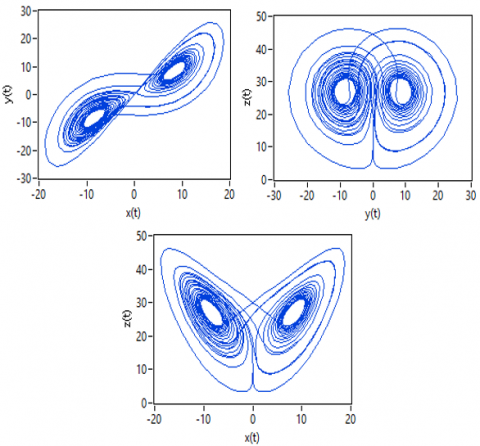Figure 1. The 2-D projections of Lorenz chaotic system on x-y, y-z, x-z plains

2.2 Sprott chaotic system

Sprott  is formed chaotic systems from A to S in 1994. While the equations between A and E have 5 terms, the equations between F and S have 6 terms. Initial conditions for case A were taken as x0=0, y0=0.5, z0=0.05. The differential equations of the Sprott chaotic system for case A are given in Eq. (2). Chaotic attractors of Sprott system are given in Figure 2.

$\dot{x}=y$

$\dot{y}=-x+y z$

$\dot{z}=1-y^{2}$               (2)

## 2.png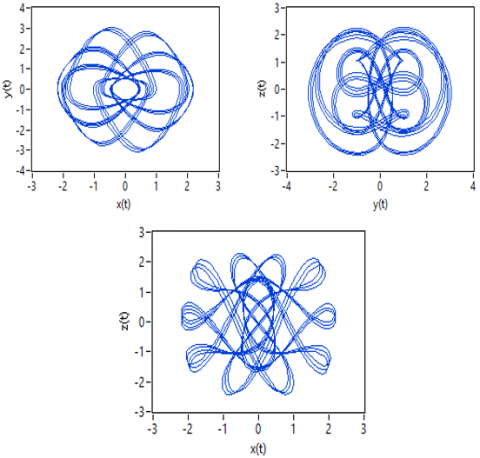Figure 2. The 2-D projections of Sprott Case A chaotic system on x-y, y-z, x-z plains

2.3 Rucklidge chaotic system

The Rucklidge chaotic system considered by Rucklidge and modelled with a third-order set of non-linear differential equations which performs chaotic outputs like chaotic Lorenz model and used frequently in fluid mechanics modelling . The differential equations of the Rucklidge chaotic system are given in Eq. (1).

$\dot{x}=-M x+L y-y z$

$\dot{y}=x$

$\dot{z}=-z+y^{2}$              (3)

M and L are positive system parameters and x, y, z are state variables. The system (3) performs chaotic behavior according to systems parameters. The 2-D projections of Rucklidge chaotic systems on (x-y) (y-z) (x-z) plains are shown Figure 3.

## 3.pngFigure 3. The 2-D projections of Rucklidge chaotic system on x-y, y-z, x-z plains

The system displays chaotic behavior when M is chosen as 2 and L is 6,7. As initial parameters when x0=1, y0=0, z0=4.5 were taken the simulation results which are in Figure 3 observed.

2.4 Moore-Spiegel chaotic system

The Moore-Spiegel system is another chaotic autonomous system which is defined by Dreek W. Moore and Spiegel  in 1965 and its state-space representation is described as in Eq. (4).

$\dot{x}=y$

$\dot{y}=z$

$\dot{z}=-z-\left(26-\tau+\tau x^{2}\right) y-26 x$              (4)

The $\tau$ parameter which is in differential equation system is 100 and when as initial conditions were taken as x0=0.1, y0=0, z0=0 chaotic results are given in Figure 4.

## 4.png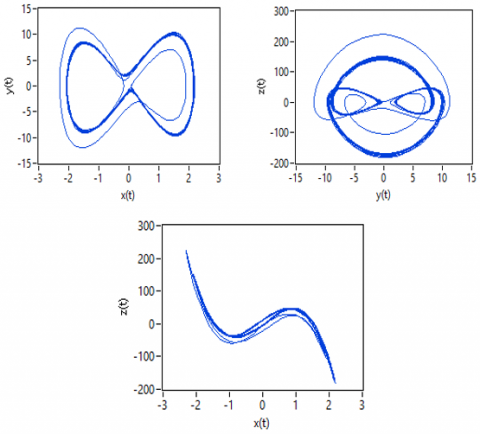Figure 4. The 2-D projections of Moore-Spiegel chaotic system on x-y, y-z, x-z plains

## 5.png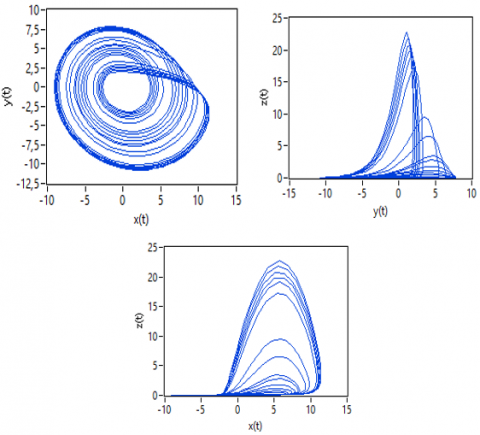Figure 5. The 2-D projections of Rössler chaotic system on x-y, y-z, x-z plains

2.5 Rössler chaotic system

The Rössler chaotic systems differential equations are shown in Eq. (5). The system parameters are a=0.2, b=0.2 and c=5.7. x, y, z are state variables and x0=-9, y0=0, z0=0 were taken as initial conditions for chaotic behavior. Differential equations of the system are given in Eq. (5). and the 3D projectors are given in Figure 5.

$\dot{x}=-y-z$

$\dot{y}=x+a y$

$\dot{z}=b+z(x-c)$                    (5)

3. Synchronzation of Chaotic Systems via Active Controller

The synchronization steps which used in the study will be explained on Rucklidge chaotic system and synchronization outputs of the five chaotic systems will be given in following section. The error variables of each output of Rucklidge chaotic systems in different initial conditions must be obtained in order to be able to perform the chaotic synchronization of the systems by the active control method . These error dynamics are obtained by subtracting the master chaotic system from the slave chaotic system. The differential equations of Master Rucklidge system in Eq. (6), Slave Rucklidge system in Eq. (7) are given. Control functions are defined as $\mu_{1}(t), \mu_{2}(t)$ and $\mu_{3}(t)$.

$\dot{x}_{m}=-M x_{m}+L y_{m}-y_{m} z_{m}$

$\dot{y}_{m}=x_{m}$

$\dot{z}_{m}=-z_{m}+y_{m}^{2}$               (6)

$\dot{x}_{s}=-M x_{s}+L y_{s}-y_{s} z_{s}+\mu_{1}(t)$

$\dot{y}_{s}=x_{s}+\mu_{2}(t)$

$\dot{z}_{s}=-z_{s}+y_{s}^{2}+\mu_{3}(t)$            (7)

The initial conditions of two chaotic system were taken as x0m=1, y0m=0, and z0m=4.5, and x0s=1.101, y0s=0.12, z0s=4.512. The error functions of these two chaotic systems are expressed in Eq. (8) and error functions are taken as e1=xs-xm, e2=ys-ym, e3=zs-zm.

$\dot{e}_{1}=-M x_{s}+M x_{m}+L y_{s}-L y_{m}-y_{s} z_{s}+y_{m} z_{m}+\mu_{1}(t)$

$\dot{e}_{2}=x_{s}-x_{m}+\mu_{2}(t)$

$\dot{e}_{3}=-z_{s}+z_{m}+y_{s}^{2}-y_{m}^{2}+\mu_{3}(t)$       (8)

As a requirement of active control method, the control functions must be selected so as to eliminate non-linear components. Since V1(t)=-k1e1, V2(t)=-k2e2, V3(t)=-k3e3 are taken in Eq. (9).

$\mu_{1}(t)=y_{s} z_{s}-y_{m} z_{m}+V_{1}(t)$

$\mu_{2}(t)=V_{2}(t)$

$\mu_{3}(t)=y_{m}^{2}-y_{s}^{2}$                (9)

Thus, the error dynamics will be turned into a linear structure and their matrix form is given below by using coefficient matrix of Eq. (8).

$\left[\begin{array}{c}\dot{e}_{1} \\ \dot{e}_{2} \\ \dot{e}_{3}\end{array}\right]=\left[\begin{array}{ccc}-\left(M+k_{1}\right) & 1 & 0 \\ L & -k_{2} & 0 \\ 0 & 0 & -\left(1+k_{3}\right)\end{array}\right]\left[\begin{array}{c}e_{1} \\ e_{2} \\ e_{3}\end{array}\right]$            (10)

Many active controller gain parameters can be selected for Rucklidge chaotic system as eigenvalues. In this study depending on the parameters of Rucklidge system k1=20, k2=30, k3=10 were selected. The master-slave synchronization of identical two Rucklidge systems is presented in Figure 6.

## 6.jpg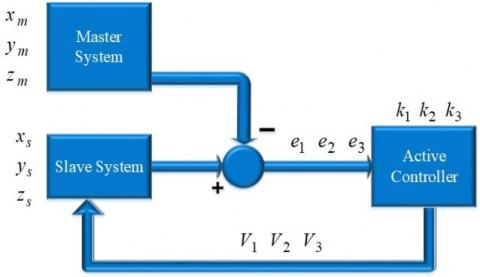Figure 6. Block diagram of master-slave synchronization

4. Experimental Work

In this chapter, simulation and real time synchronization of chaotic systems within LabVIEW environment was achieved by using controller parameters so that performing the Master-Slave synchronization. Master and Slave parts of each chaotic system were established in LabVIEW block diagram and simulation results were achieved in front panel. The simulation was performed for 30 seconds and the controller was activated in 20th second. It was observed that the slave system followed the master system shortly after the active controller switched on.

The chaotic attractors of each master-slave chaotic system and the error between them were projected. Error graphs for each state variable, 2-D phase plains and xm-xs ym-ys zm-zs diagrams were projected on a single chart in order to ensure the synchronization which occurred in 20th second. In the system that was created to observe 5 different chaotic system outputs on a single graph after synchronization status, each chaotic system was numbered between 0-4. When the number of the system to be watched entered in the indicator part of the panel, the outputs of that system were observed. Output results of each chaotic systems are given below.

NI-6009 DAQ card used for real time application of chaotic systems. In this application since the analog output of the DAQ card can only generate a voltage between 0 and 5 volts, the outputs of the state variables were weakened according to the need and DC signal component was added for the purpose of preventing signal to go to negative. NI-6009 DAQ card and real time application experimental setup are given in Figure 7 and Figure 8.

Real-time oscilloscope images for each chaotic system as 2D are given in Figure 9.

In addition to all these studies, secure communication simulation was performed on Lorenz chaotic system with master-slave synchronization and also and image encryption application was performed using Chen chaotic system. A sinusoidal signal (0.2 Hz frequency / 0.1V amplitude) used as data signal. The data signal was modulated with the signal taken from master chaotic system after the transmitted signal demodulated by the slave chaotic system . The simulation projections of data signal, modulated signal and demodulated signal are given in Figure 10.

## 7.jpg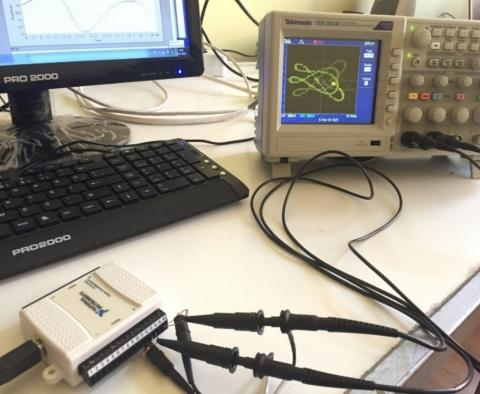Figure 7. NI-6009 DAQ card

## 8.jpg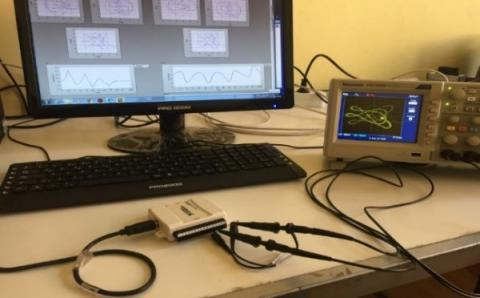Figure 8. Real-time LabVIEW based application

## 9.pngFigure 9. Chaotic attractors Lorenz, Sprott, Rucklidge Moore-Spiegel, Rössler

To perform image encryption, an image which is desired to be encrypted between Chen master and slave chaotic systems was first encrypted with master system state variables and then decrypted with slave system state variables. Image encryption simulation was performed for 30 seconds. After sampling the Xm, Ym, Zm and Xs, Ys, Zs chaotic signals which were acquired from master and slave systems separately for 30 seconds scaled according to the desired image information format to be transmitted. The image encryption was realized by performing XOR processing between image data and scaled state variables data which was sampled for 30 seconds. Decryption was also realized with XOR processing between encrypted data and Xs Ys Zs state variables. Then by 20th second decryption was realized with second XOR operation since the slave systems state variables Xs Ys Zs were equal to the master system Xm Ym Zm state variables. As a result of all these operations, the original image was obtained [33-36]. Encryption of the image was performed to sample images and real time application results are shown in the Figure 11, 12 and 13.

In order to see how prosperous, the encryption process was, the histograms of the original grayscale image and the encrypted image were examined and when the histogram graph analyzed, it appeared that there was very little affinity between the encrypted image and the original image which means that the encryption process performed successfully. The histogram graph of original and encrypted image for Lena is given in Figure 14.

## 10a.png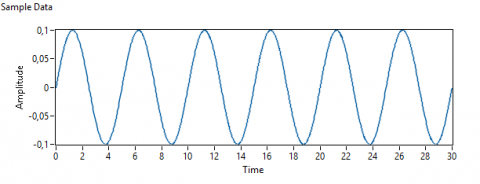## 10b.png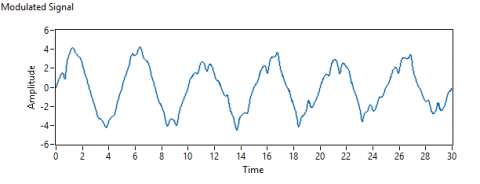## 10c.png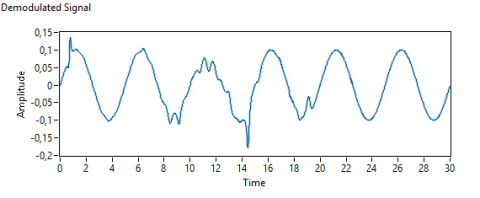Figure 10. Simulated graphs of data signal modulated signal and demodulated Signal

## 11.png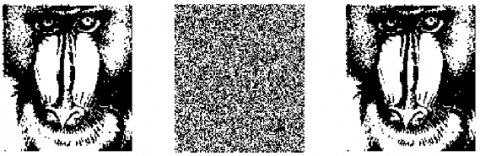Figure 11. Baboon (Binary) Image (a) Input Image (b) Encrypted Image (c) Decrypted Image

## 12.pngFigure 12. Baboon (RGB) Image (a) Input Image (b) Encrypted Image (c) Decrypted Image

## 13.pngFigure 13. Lena (RGB) Image (a) Input Image (b) Encrypted Image (c) Decrypted Image

## 14.png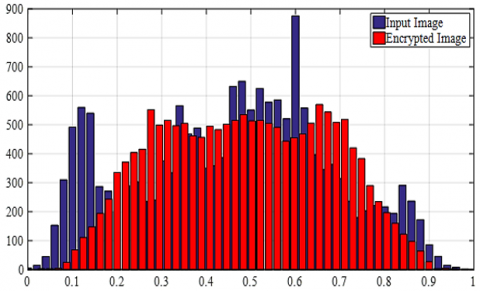Figure 14. Histogram graph of input and encrypted Lena image

Other similarity parameters such as correlation coefficient, peak signal to noise ratio (PSNR) and structural similarity index (SSIM) between the original image and the encrypted image examined in addition to the histogram graph used to test the similarity. The correlation coefficient is a 2-dimensional coefficient and varies between -1 and 1 [37, 38]. As the similarity between the images increases, this coefficient approaches to 1 and if the similarity decreases the coefficient approaches -1. On the other hand, the SSIM parameter, which is another structural similarity index, takes values between 0 and 1, and the similarity between the images increases as this parameter approaches 1 . PSNR coefficient is useful for determining the similarity between two images .

The similarity parameters obtained in the study are shown in the Table 1. When the table was examined, it was seen that these similarity parameters between the original image and the encrypted image were obtained optimally which means an appropriate encryption was performed.

Table 1. Similarity measurement parameters

 Parameters Between input and encrypted image Correlation Coefficient 0.0031 PSNR 10.7576 SSIM 0.0294
5. Conclusions

So far although there are many studies on chaotic system simulation and synchronization in the literature, there are very few studies involving real-time applications. Real-time applications will be easier to implement on chaotic systems and the number of these studies will increase with the development of programs that incorporate graphic-based software and hardware systems such as LabVIEW. In this paper the master-slave synchronization of five different chaotic systems was achieved via active controller method. Synchronization simulated in LabVIEW environment and it was observed that the slave system followed the master system after controller activated for all three state variables.

The error between the systems went zero after the activation of controller as well. Chaotic attractors and error graphics of five different chaotic systems were displayed as real time on an oscilloscope by DAQ card. Also, a secure communication simulation and image encryption application were performed on the Lorenz and Chen chaotic systems successfully. As a result, synchronization and secure communication were performed as real time in LabVIEW environment and according to the obtained experimental results, it was seen that LabVIEW environment provided substantial convenience for real time implementation of chaotic systems and for its applications.

References

 Saini, I., Singh, P., Yadav, A.K. (2017). Analysis of Lorenz-chaos and exclusive-OR based image encryption scheme. International Journal of Social Computing and Cyber-Physical Systems, 2(1): 59-72. https://doi.org/10.1504/IJSCCPS.2017.088769

 Sprott, J.C. (1994). Some simple chaotic flows. Physical Review E, 50(2): R647. https://doi.org/10.1103/PhysRevE.50.R647

 Rucklidge, A.M. (1992). Chaos in models of double convection. Journal of Fluid Mechanics, 237: 209-229. https://doi.org/10.1017/S0022112092003392

 Moore, D.W., Spiegel, E.A. (1966). A thermally excited non-linear oscillator. The Astrophysical Journal, 143: 871.

 Laiphrakpam, D.S., Khumanthem, M.S. (2017). Cryptanalysis of symmetric key image encryption using chaotic Rossler system. Optik, 135: 200-209. https://doi.org/10.1016/j.ijleo.2017.01.062

 Pecora, L.M., Carroll, T.L. (1990). Synchronization in chaotic systems. Physical Review Letters, 64(8): 821. https://doi.org/10.1103/PhysRevLett.64.821

 Kocarev, L., Parlitz, U. (1995). General approach for chaotic synchronization with applications to communication. Physical Review Letters, 74(25): 5028. https://doi.org/10.1103/PhysRevLett.74.5028

 Xie, Q., Chen, G., Bollt, E.M. (2002). Hybrid chaos synchronization and its application in information processing. Mathematical and Computer Modelling, 35(1-2): 145-163. https://doi.org/10.1016/S0895-7177(01)00157-1

 Liao, T.L. (1998). Adaptive synchronization of two Lorenz systems. Chaos, Solitons & Fractals, 9(9): 1555-1561. https://doi.org/10.1016/S0960-0779(97)00161-6

 Boccaletti, S., Valladares, D.L. (2000). Characterization of intermittent lag synchronization. Physical Review E, 62(5): 7497. https://doi.org/10.1103/PhysRevE.62.7497

 Rosenblum, M.G., Pikovsky, A.S., Kurths, J. (1997). From phase to lag synchronization in coupled chaotic oscillators. Physical Review Letters, 78(22): 4193. https://doi.org/10.1103/PhysRevLett.78.4193

 Alasty, A., Salarieh, H. (2005). Controlling the chaos using fuzzy estimation of OGY and Pyragas controllers. Chaos, Solitons & Fractals, 26(2): 379-392. https://doi.org/10.1016/j.chaos.2004.12.034

 Roy, A., Misra, A.P., Banerjee, S. (2019). Chaos-based image encryption using vertical-cavity surface-emitting lasers. Optik, 176: 119-131. https://doi.org/10.1016/j.ijleo.2018.09.062

 Wu, Z., Zhang, X., Zhong, X. (2019). Generalized chaos synchronization circuit simulation and asymmetric image encryption. IEEE Access, 7: 37989-38008. https://doi.org/10.1109/ACCESS.2019.2906770

 Yeh, J.P., Wu, K.L. (2008). A simple method to synchronize chaotic systems and its application to secure communications. Mathematical and Computer Modelling, 47(9-10): 894-902. https://doi.org/10.1016/j.mcm.2007.06.021

 Lu, S., Chen, L. (2008). A general synchronization method of chaotic communication systems via Kalman filtering. Kybernetika, 44(1): 43-52. http://dml.cz/dmlcz/135832

 Wang, Q.Y., Lu, Q.S., Chen, G.R., Guo, D.H. (2006). Chaos synchronization of coupled neurons with gap junctions. Physics Letters A, 356(1): 17-25. https://doi.org/10.1016/j.physleta.2006.03.017

 Haeri, M., Khademian, B. (2006). Comparison between different synchronization methods of identical chaotic systems. Chaos, Solitons & Fractals, 29(4): 1002-1022. https://doi.org/10.1016/j.chaos.2005.08.101

 Chen, Y.J., Chou, H.G., Wang, W.J., Tsai, S.H., Tanaka, K., Wang, H.O., Wang, K.C. (2020). A polynomial-fuzzy-model-based synchronization methodology for the multi-scroll Chen chaotic secure communication system. Engineering Applications of Artificial Intelligence, 87: 103251. https://doi.org/10.1016/j.engappai.2019.103251

 Grzybowski, J.M.V., Rafikov, M., Balthazar, J.M. (2009). Synchronization of the unified chaotic system and application in secure communication. Communications in Nonlinear Science and Numerical Simulation, 14(6): 2793-2806. https://doi.org/10.1016/j.cnsns.2008.09.028

 Sadoudi, S., Azzaz, M. S., Djeddou, M., Benssalah, M. (2009). An FPGA real-time implementation of the Chen’s chaotic system for securing chaotic communications. International Journal of Nonlinear Science, 7(4): 467-474.

 Koyuncu, I., Ozcerit, A.T., Pehlivan, I. (2014). Implementation of FPGA-based real time novel chaotic oscillator. Nonlinear Dynamics, 77(1-2): 49-59. https://doi.org/10.1007/s11071-014-1272-x

 Rodriguez-Bollain, A., Mata-Machuca, J.L., Martinez-Guerra, R. (2010). Synchronization of chaotic systems: A real-time application to Colpitts oscillator. In 2010 7th International Conference on Electrical Engineering Computing Science and Automatic Control, pp. 60-65. IEEE. https://doi.org/10.1109/ICEEE.2010.5608584

 Azzaz, M.S., Tanougast, C., Sadoudi, S., Bouridane, A., Dandache, A. (2010). An FPGA implementation of a Feed-Back Chaotic Synchronization for secure communications. In 2010 7th International Symposium on Communication Systems, Networks & Digital Signal Processing (CSNDSP 2010), pp. 239-243. https://doi.org/10.1109/CSNDSP16145.2010.5580426

 Bulut, G.G., Sahin, M.E., Guler, H. (2018). An implementation of chaotic circuits with multisim-LabVIEW. International Advanced Researches and Engineering Journal, 304-308.

 Astakhov, V.V., Anishchenko, V.S., Kapitaniak, T., Shabunin, A.V. (1997). Synchronization of chaotic oscillators by periodic parametric perturbations. Physica D: Nonlinear Phenomena, 109(1-2): 11-16. https://doi.org/10.1016/S0167-2789(97)00153-X

 Blazejczyk-Okolewska, B., Brindley, J., Czolczynski, K., Kapitaniak, T. (2001). Antiphase synchronization of chaos by noncontinuous coupling: Two impacting oscillators. Chaos, Solitons & Fractals, 12(10): 1823-1826. https://doi.org/10.1016/S0960-0779(00)00145-4

 Yang, X.S., Duan, C.K., Liao, X.X. (1999). A note on mathematical aspects of drive–response type synchronization. Chaos, Solitons & Fractals, 10(9): 1457-1462. https://doi.org/10.1016/S0960-0779(98)00123-4

 Lü, J., Zhang, S. (2001). Controlling Chen's chaotic attractor using backstepping design based on parameters identification. Physics Letters A, 286(2-3): 148-152. https://doi.org/10.1016/S0375-9601(01)00383-8

 Gupta, J., Kosta, S.P., Mor, P. (2011). Simulation of non-autonomous chaotic circuit on LabVIEW using nonlinear electrolytic device. Int. J. of Electronics Engineering, 3(2): 215-220.

 Bai, E.W., Lonngren, K.E. (1997). Synchronization of two Lorenz systems using active control. Chaos, Solitons & Fractals, 8(1): 51-58. https://doi.org/10.1016/S0960-0779(96)00060-4

 Guler, H., Celik, V., Kaya, T., Erol, Y. (2018). The real time implementation of a chaotic system’s synchronization for secure communication. Tehnički Vjesnik, 25: 43-48. https://doi.org/10.17559/TV-20160420113930

 Dhall, S., Pal, S.K., Sharma, K. (2018). Cryptanalysis of image encryption scheme based on a new 1D chaotic system. Signal Processing, 146: 22-32. https://doi.org/10.1016/j.sigpro.2017.12.021

 Diaconu, A.V. (2016). Circular inter–intra pixels bit-level permutation and chaos-based image encryption. Information Sciences, 355: 314-327. https://doi.org/10.1016/j.ins.2015.10.027

 Wang, B., Zou, F.C., Cheng, J. (2018). A memristor-based chaotic system and its application in image encryption. Optik, 154: 538-544. https://doi.org/10.1016/j.ijleo.2017.10.080

 Lan, R., He, J., Wang, S., Gu, T., Luo, X. (2018). Integrated chaotic systems for image encryption. Signal Processing, 147: 133-145. https://doi.org/10.1016/j.sigpro.2018.01.026

 Catalbas, M.C., Gulten, A. (2018). A novel super resolution approach for computed tomography images by inverse distance weighting method. Journal of the Faculty of Engineering and Architecture of Gazi University 33(2): 671-684. https://doi.org/10.17341/gazimmfd.416379

 Cai, Q.R. (2019). A secure image encryption algorithm based on composite chaos theory. Traitement du Signal, 36(1) : 31-36. https://doi.org/10.18280/ts.360104

 Ma, K., Duanmu, Z., Yeganeh, H., Wang, Z. (2017). Multi-exposure image fusion by optimizing a structural similarity index. IEEE Transactions on Computational Imaging, 4(1): 60-72. https://doi.org/10.1109/TCI.2017.2786138

 Huang, Y., Niu, B., Guan, H., Zhang, S. (2019). Enhancing image watermarking with adaptive embedding parameter and PSNR guarantee. IEEE Transactions on Multimedia, 21(10): 2447-2460. https://doi.org/10.1109/TMM.2019.2907475